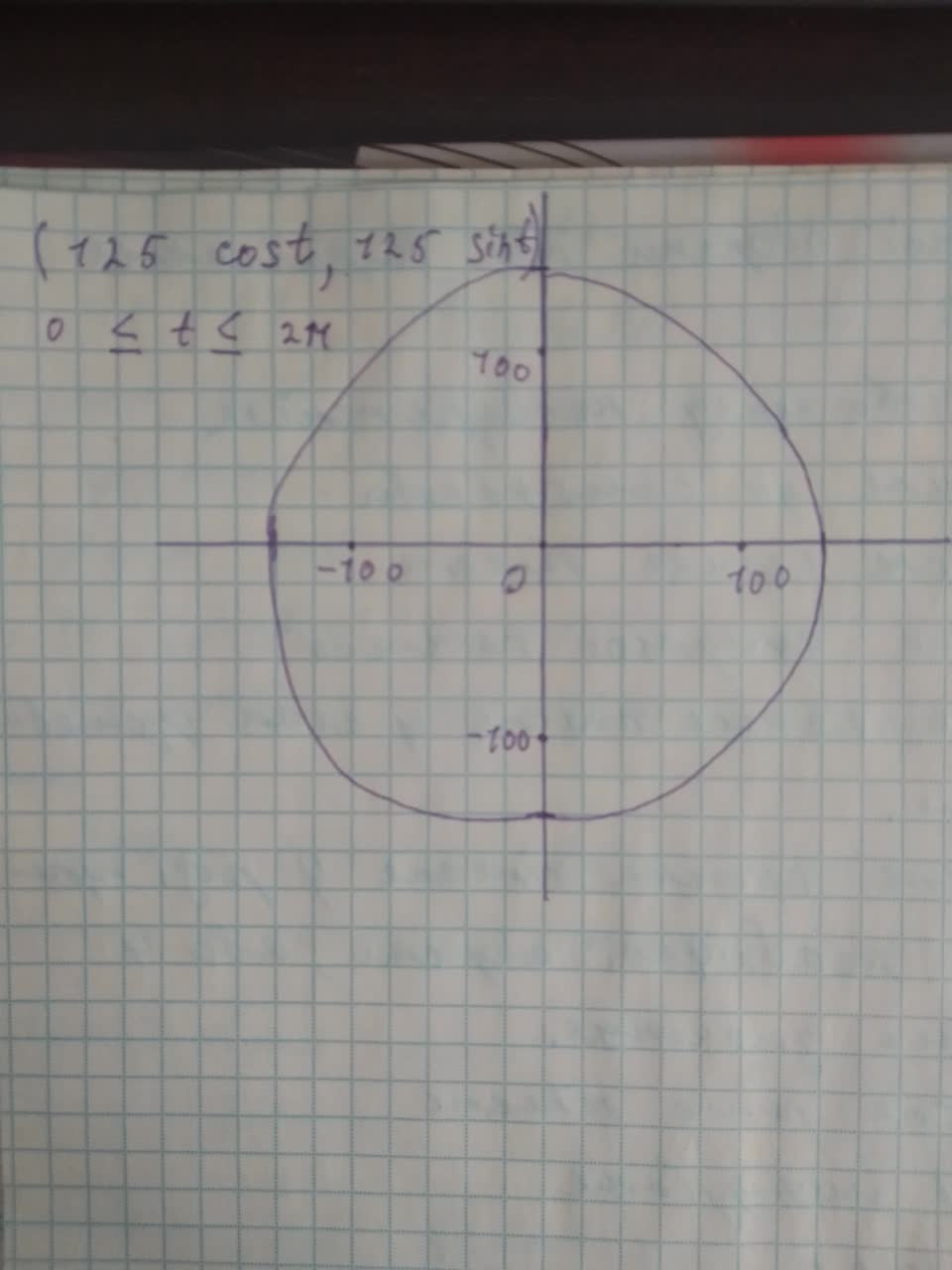# Graph the plane curve defined by the parametric equationsx = 125 cos t and y = 125 sin tbeljuA 2021-02-24 Answered

Graph the plane curve defined by the parametric equations $$x = 125 \cos t\ and\ y = 125 \sin t$$

• Questions are typically answered in as fast as 30 minutes

### Plainmath recommends

• Get a detailed answer even on the hardest topics.
• Ask an expert for a step-by-step guidance to learn to do it yourself.Tasneem Almond

Step 1 We have to graph the plane curve defined by the parametric equations $$x=125 \cos t\ and\ y=125 \sin t$$

Step 2 The parametric equations defined by $$x=125 \cos\ t\ and\ y=125$$sintrepresents the equation of a circle with center at origin and radius 125. Now ,We graph the parametric equation defined by $$x=125 \cos\ t\ and\ y = 125\sin t, 0 \leq t \leq 2\pi$$